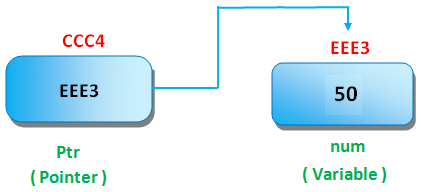# Pointer in C

## Pointer in C

A pointer is a variable which contains or hold the address of another variable. We can create pointer variable of any type of variable for example integer type pointer is 'int *ptr'.

In pointer following symbols are use;

SymbolNmeDescription
* (asterisk sign)Indirection operatorGives the contents of an object pointed to by a pointer.

• Pointer reduces the code and improves the performance, because it direct access the address of variable.
• Using pointer concept we can return multiple value from any function.
• Using pointer we can access any memory location from pointer.

The address of operator & gives the address of a variable. For display address of variable, we need %u.

## Example

```#include<stdio.h>
void main()
{
int a=50;
printf("\nValue of a is: %d",n);
}
```

## Output

```Value of a is: 50
```

## Declaring a pointer

In C language for declared pointer we can use * (asterisk symbol).

## Syntax

```int *p;  //pointer to integer
char *ch; //pointer to character
```

## Example of pointer

In below image pointer variable stores the address of num variable i.e. EEE3. The value of num is 50 and address of pointer prt is CCC4## Example

```#include<stdio.h>
int main ()
{
int num=50;
int  *ptr; // pointer variable
ptr = # // store address of variable in pointer
printf("Address of num variable: %x\n", &num);
/* address stored in pointer variable */
printf("Address stored in ptr variable: %x\n", ptr );
/* access the value using the pointer */
printf("Value of *ptr variable: %d\n", *ptr );
return 0;
}
```

## Output

```Address of num variable: EEE3
Address stored in ptr variable: CCC4
Value of *ptr variable: 50
```

## NULL Pointer

A pointer that is not assigned any value but NULL is known as NULL pointer. This is done at the time of variable declaration. The NULL pointer is a constant which is defined in standard libraries with zero value.

## Example

```#include<stdio.h>
int main ()
{
int  *ptr = NULL;
printf("Value of ptr is: %x", ptr  );
return 0;
}
```

## Output

```Value of ptr is: 0
```